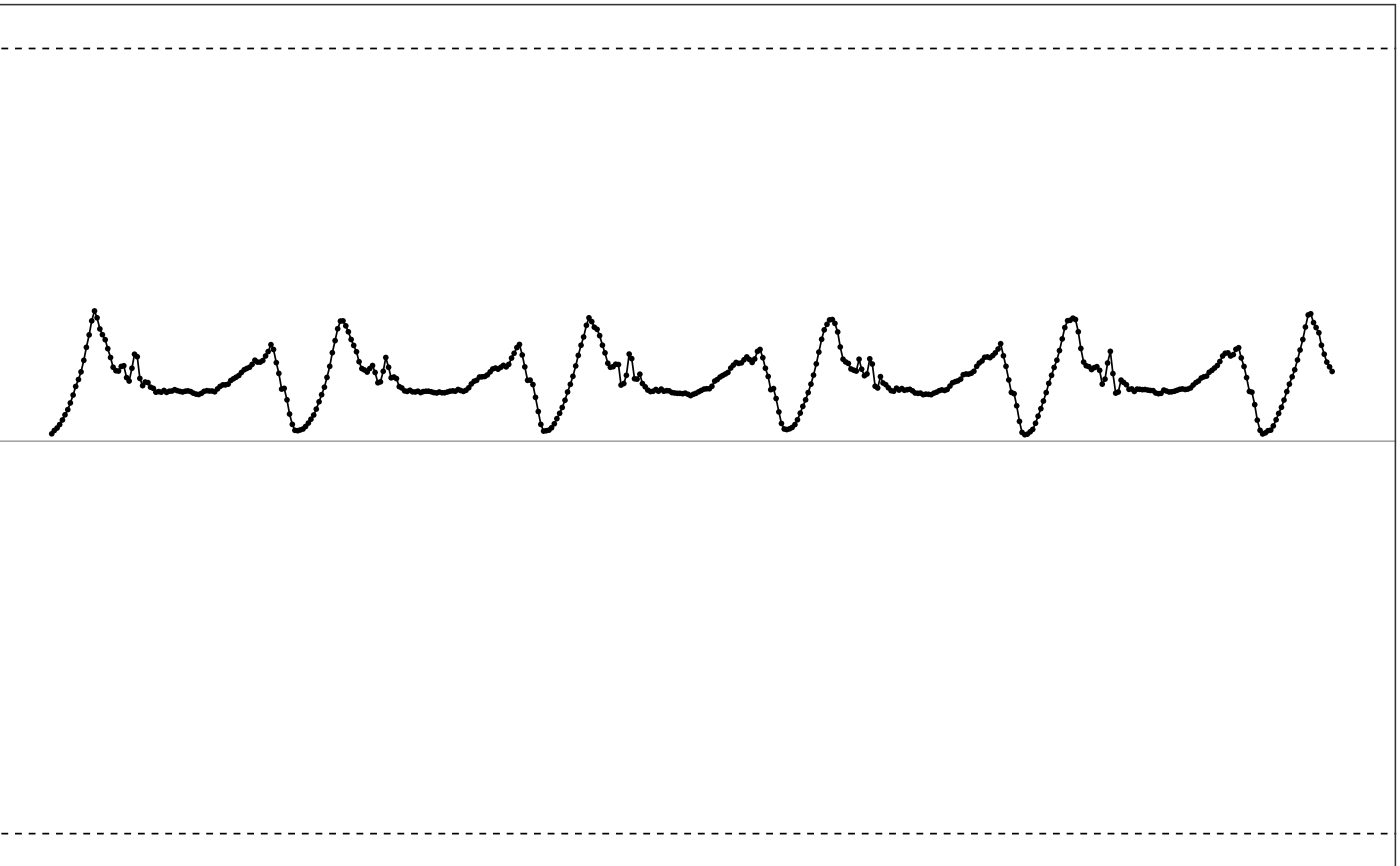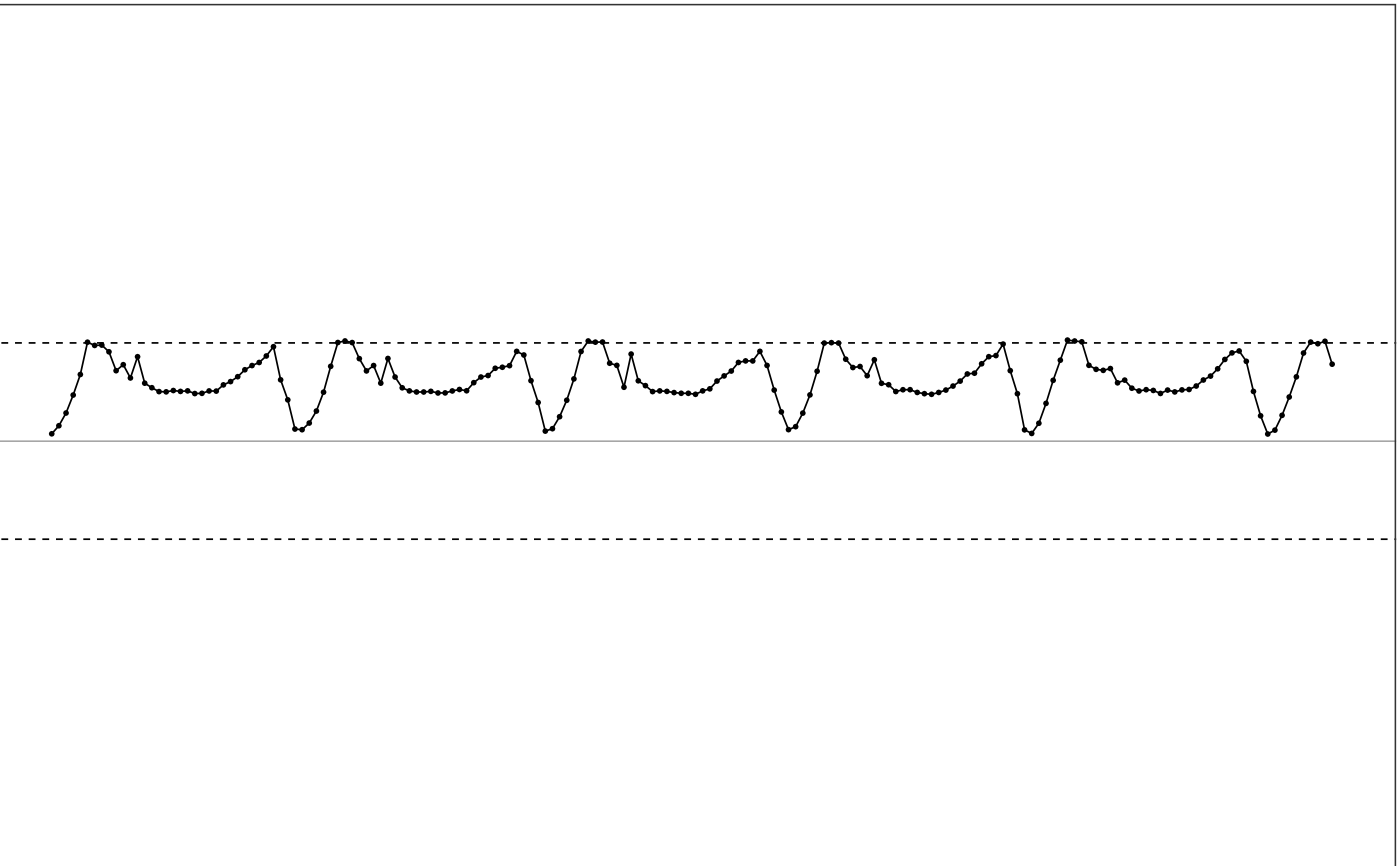simulate_new_data simulate new data based on the given multi-channel accelerometer data, a new dynamic range and a new sampling rate.

simulate_new_data(old_data, new_range, new_sr)

## Arguments

old_data dataframe. Input multi-channel accelerometer data. numerical vector. The new dynamic ranges to cut off the signal. Should be a 2-element numerical vector. c(low, high), where low is the negative max value the device can reach and high is the positive max value the device can reach. Default is NULL, meaning the function will do nothing but return the input data. number. New sampling rate in Hz.

## Details

This function simulates the data from a new device based on the signal from a baseline device. It first changes the sampling rate using function interpolate_signal, and then changes the dynamic range using function cut_off_signal.

## How is it used in MIMS-unit algorithm?

This function is a utility function that is used to simulate new devices with different sampling rates and dynamic ranges during algorithm validation.

Other utility functions: clip_data(), cut_off_signal(), interpolate_signal(), parse_epoch_string(), sampling_rate(), segment_data()

## Examples

  # Use sample data for testing
df = sample_raw_accel_data

# Show df
illustrate_signal(df, range=c(-8, 8))# simulate new data by changing range and sampling rate
new_df = simulate_new_data(df, new_range=c(-2, 2), new_sr = 30)

# Show new df
illustrate_signal(new_df, range=c(-2, 2))